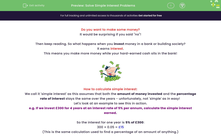# Solve Simple Interest Problems

In this worksheet, students will calculate simple interest, which assumes that the amount of money invested and the percentage rate of interest stays the same over time.Key stage:  KS 4

Year:  GCSE

GCSE Subjects:   Maths

GCSE Boards:   AQA, Eduqas, Pearson Edexcel, OCR,

Curriculum topic:   Ratio, Proportion and Rates of Change

Curriculum subtopic:   Ratio, Proportion and Rates of Change Discrete Growth and Decay

Popular topics:   Money worksheets

Difficulty level:#### Worksheet Overview

Do you want to make some money?

It would be surprising if you said "no"!

Then keep reading. So what happens when you invest money in a bank or building society?

It earns interest.

This means you make more money while your hard-earned cash sits in the bank!How to calculate simple interest:

We call it 'simple interest' as this assumes that both the amount of money invested and the percentage rate of interest stays the same over the years - unfortunately, not 'simple' as in easy!

Let's look at an example to see this in action.

e.g. If we invest £300 for 4 years at an interest rate of 5% per annum, calculate the simple interest earned.

So the interest for one year is 5% of £300:

300 × 0.05 = £15

(This is the same calculation used to find a percentage of an amount of anything.)

Now we have £15 at the end of the year to go spend, and we haven't had to do a thing!

Assuming we take our £15 at the end of each year, how much will we have at the end of 4 years?

£15 × 4 = £60

Remember, we have not invested any more money, and the interest rate has stayed the same.We are now going to raid the piggy bank and put our money in the building society!

If we invest £400 for 1.5 years at an interest rate of 6.5%, how much simple interest will we earn?

Interest for a year = 400 × 0.065 = £26

Interest for 6 months = £26 ÷ 2 = £13

Interest for 1.5 years = £26 + £13 = £39Sometimes people take a fall as they don't understand simple interest.

If we are going to borrow money, we don't earn interest, we have to pay it instead!

The interest is calculated in the exact same way, but we will be owing money to the bank in this scenario.

In this activity, we will calculate simple interest using the method above, which assumes that the amount of money invested and the percentage rate of interest stays the same over time.

### What is EdPlace?

We're your National Curriculum aligned online education content provider helping each child succeed in English, maths and science from year 1 to GCSE. With an EdPlace account you’ll be able to track and measure progress, helping each child achieve their best. We build confidence and attainment by personalising each child’s learning at a level that suits them.

Get started#### Popular Maths topics

••••